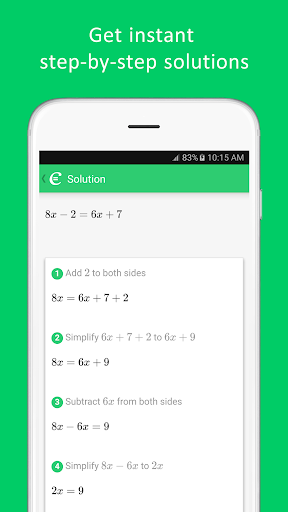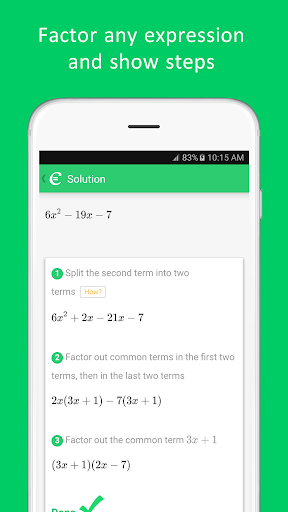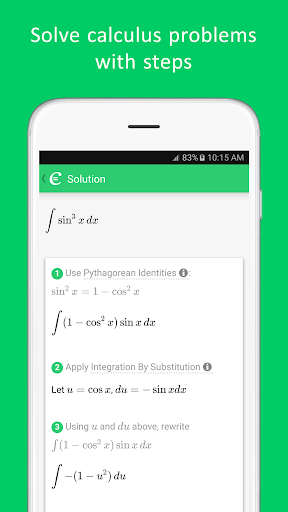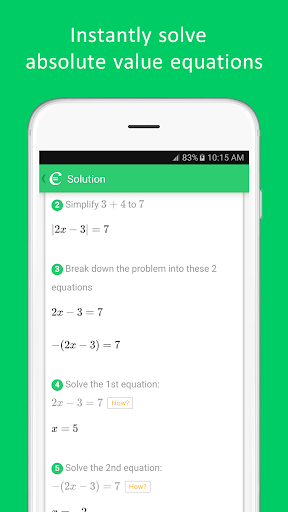# Cymath - Math Problem Solver

##### About Cymath - Math Problem Solver
With millions of Cymath.com users worldwide, the Cymath math problem solver app uses the same math engine while letting you solve problems on the go! Just enter a problem from your math homework, and let Cymath solve it for you step-by-step! We provide algebra as well as calculus help.